# Assertion & Reason Test: Polynomials - 1

## 10 Questions MCQ Test Online MCQ Tests for Class 10 | Assertion & Reason Test: Polynomials - 1

Description
Attempt Assertion & Reason Test: Polynomials - 1 | 10 questions in 20 minutes | Mock test for Class 10 preparation | Free important questions MCQ to study Online MCQ Tests for Class 10 for Class 10 Exam | Download free PDF with solutions
QUESTION: 1

### Direction: In the following questions, a statement of assertion (A) is followed by a statement of reason (R). Mark the correct choice as:Assertion : If α,β, γ are the zeroes of x3 - 2x2 + qx - r and α + β = 0 , then 2q = r.Reason : If α,β, γ are the zeroes of ax3 + bx2 + cx + d , then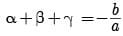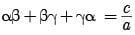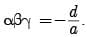Solution:

Clearly, Reason is true. [Standard Result]

α + β + γ = -(- 2) = 2

0 + γ = 2

γ = 2

αβγ = -(- r) = r

αβ(2) = r

αβ = r/2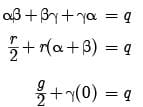γ = 2q Assertion is true.

Since, Reason gives Assertion.

QUESTION: 2

### Direction: In the following questions, a statement of assertion (A) is followed by a statement of reason (R). Mark the correct choice as:Assertion : P(x) = 4x3 – x2 + 5x4 + 3x – 2 is a polynomial of degree 3.Reason : The highest power of x in the polynomial P(x) is the degree of the polynomial.

Solution: The highest power of x in the polynomial P(x) = 4x3 – x2 + 5x4 + 3x – 2 is 4.

Therefore, the degree of the polynomial P(x) is 4.

QUESTION: 3

### Direction: In the following questions, a statement of assertion (A) is followed by a statement of reason (R). Mark the correct choice as:Assertion (A): If the zeroes of a quadratic polynomial ax2 + bx + c are both positive, then a, b and c all have the same sign.Reason (R): If two of the zeroes of a cubic polynomial are zero, then it does not have linear and constant terms.

Solution: Let α and β be the roots of the quadratic polynomial. If α and β are positive then α + β = -b/a it it shows that -b/a is negative but sum of two positive numbers (α, β) must be +ve i.e., either b or a must be negative. So, a, b and c will have different signs.

∴ Given statement is incorrect.

In case of reason:

Let β = 0, γ = 0

f(x) = (x – α) (x – β) (x – γ)

= (x – α) x · x

⇒ f(x) = x3 – αx2 which has no linear (coefficient of x) and constant terms.

∴ Given statement is correct.

Thus, assertion is incorrect but reason is correct.

QUESTION: 4

Direction: In the following questions, a statement of assertion (A) is followed by a statement of reason (R). Mark the correct choice as:

Assertion : (2 - √3) is one zero of the quadratic polynomial then other zero will be (2 + √3).

Reason : Irrational zeros (roots) always occurs in pairs.

Solution: As irrational roots/zeros always occurs in pairs therefore, when one zero is (2 - √3) then other will be (2 + √3). So, both A and R are correct and R explains A.
QUESTION: 5

Direction: In the following questions, a statement of assertion (A) is followed by a statement of reason (R). Mark the correct choice as:

Assertion : x3 + x has only one real zero.

Reason : A polynomial of nth degree must have n real zeroes.

Solution: Reason is false [a polynomial of nth degree has at most n zeroes.] Again, x3 + x = x(x2 + 1) which has only one real zero i.e. x = 0

[x2 + 1 ≠ 0 for all x ∈ R]

QUESTION: 6

Direction: In the following questions, a statement of assertion (A) is followed by a statement of reason (R). Mark the correct choice as:

Assertion (A): The value of k for which the quadratic polynomial kx2 + x + k has equal zeroes are ± 1/2.

Reason (R): If all the three zeroes of a cubic polynomial x3 + ax2 – bx + c are positive, then at least one of a, b and c is non-negative.

Solution: In case of assertion

f(x) = kx2 + x + k (a = k, b = 1, c = k)

For equal roots b2 – 4ac = 0

⇒ (1)2 – 4(k) (k) = 0

⇒ 4k2 = 1

⇒ k2 = 1/4

⇒ k = ± 1/2

So, there are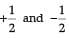values of k so that

the given equation has equal roots.

∴ Given statement is correct.

In case of reason:

All the zeroes of cubic polynomial are positive only when all the constants a, b, and c are negative.

∴ Given statement is incorrect.

Thus, assertion is correct but reason is incorrect.

QUESTION: 7

Direction: In the following questions, a statement of assertion (A) is followed by a statement of reason (R). Mark the correct choice as:
Assertion : Zeroes of f(x) = x2 - 4x - 5 are 5, - 1
Reason : The polynomial whose zeroes are 2 + √3, 2 - √3 is x2 - 4x + 7.

Solution:

Zeroes of f(x) = x2 − 4x − 5 are obtained by solving:
x2 − 4x − 5 = 0, which implies
x2 − 5x + x − 5 = 0 or, x(x − 5) + 1(x − 5) = 0
which means x = 5 or x = −1
Thus the assertion is correct.
However, the reason is incorrect.
The numbers given are not the zeroes  of x2 − 4x + 7

QUESTION: 8

Direction: In the following questions, a statement of assertion (A) is followed by a statement of reason (R). Mark the correct choice as:

Assertion : If one zero of poly-nominal p(x) = (k2 + 4)x2 + 13x + 4k is reciprocal of other, then k = 2.

Reason : If (x – a) is a factor of p(x), then p(a) = 0 i.e. a is a zero of p(x).

Solution: Let α, 1/α be the zeroes of p(x) then we have

Product of Zeroes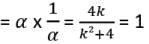⇒ k2 – 4k + 4 = 0

⇒ (k – 2)2 = 0 ⇒ k = 2

QUESTION: 9

Direction: In the following questions, a statement of assertion (A) is followed by a statement of reason (R). Mark the correct choice as:

Assertion (A): The graph of y = p(x), where p(x) is a polynomial in variable x, is as follows: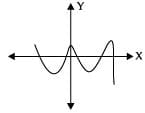The number of zeroes of p(x) is 5.

Reason (R): If the graph of a polynomial intersects the x-axis at exactly two points, it need not be a quadratic polynomial.

Solution: In case of assertion: Since the graph touches the x-axis 5 times, So, the number of zeroes of p(x) is 5.

∴ Given statement is correct.

In case of reason:

If a polynomial of degree more than two has two real zeroes and other zeroes are not real or are imaginary, and then graph of the polynomial will intersect at two points on x-axis.

∴ Given statement is correct:

Thus, both assertion and reason are correct but reason is not the correct explanation for assertion.

QUESTION: 10

Direction: In the following questions, a statement of assertion (A) is followed by a statement of reason (R). Mark the correct choice as:

Assertion : x2 + 4x + 5 has two zeroes.

Reason : A quadratic polynomial can have at the most two zeroes.

Solution: Assertion: Consider the given polynomial x 2 + 4x + 5

Because the degree of the polynomial is 2. It is a quadratic polynomial. We know that the quadratic polynomial has at the most two zeroes.

∴ x2 + 4x + 5 has two zeroes.

∴ Assertion is true.

Reason: Clearly, Reason is true.

Since both the Assertion and Reason are true and Reason is a correct explanation of Assertion.Use Code STAYHOME200 and get INR 200 additional OFF Use Coupon Code